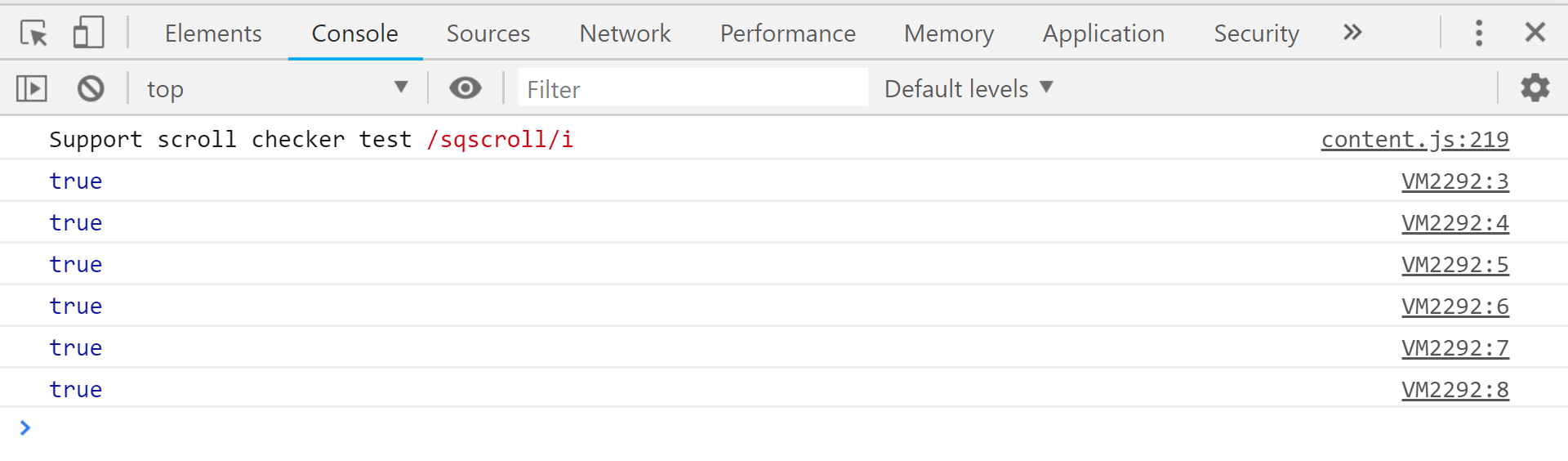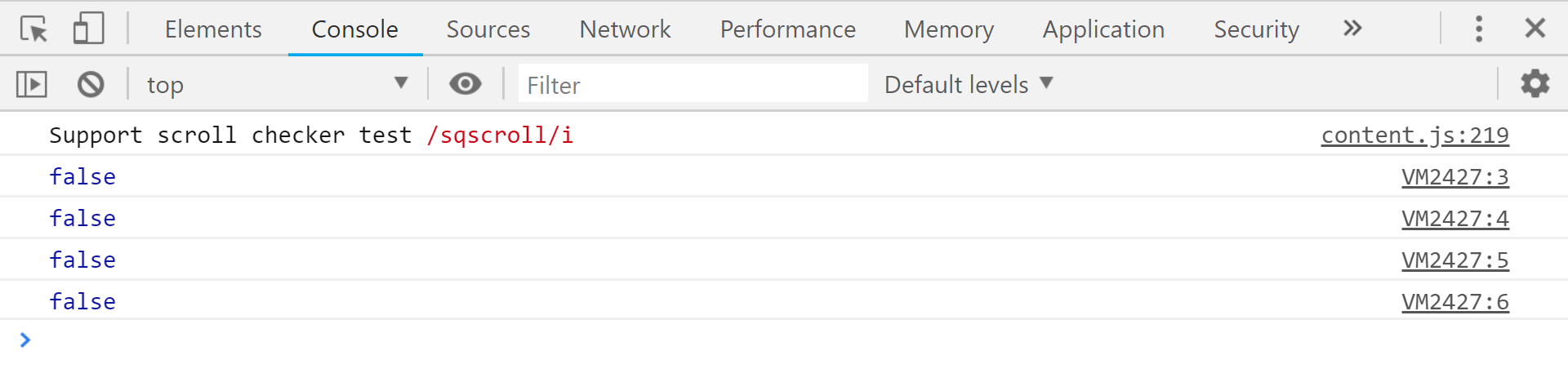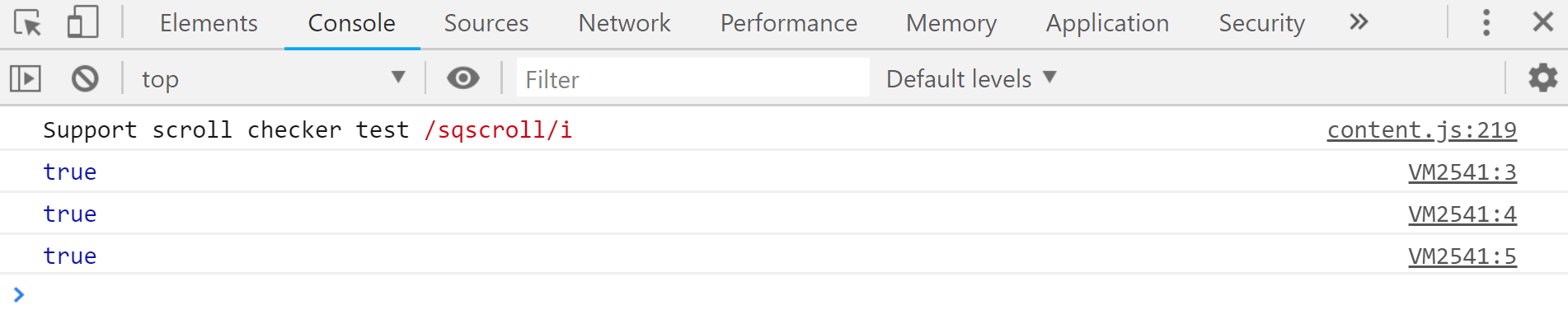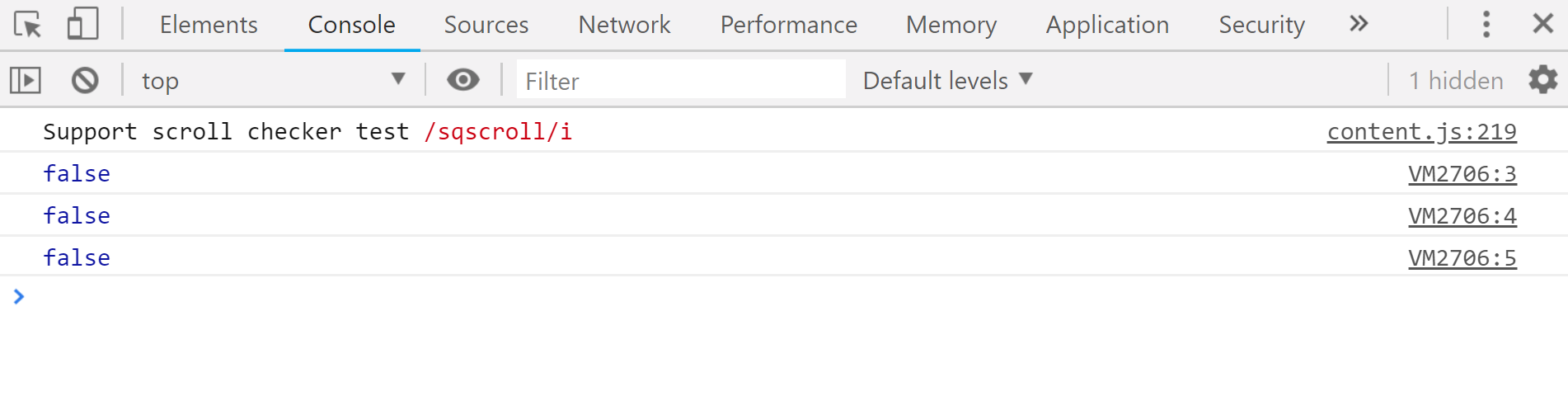# JavaScript | ‘===’ vs ‘==’Comparison Operator

In Javascript(ES6), there are four ways to test equality which are listed below:

• Using ‘==’ operator
• Using ‘===’ operator
• SameValueZero: used mainly in sets, maps and arrays.
• SameValue: used elsewhere

Now, our main concern is getting to know the difference between the ‘==’ and ‘===’ operators that the javascript provides, though they look similar, yet they are very different.

‘==’ operator: In Javascript, the ‘==’ operator is also known as loose equality operator which is mainly used to compare two value on both the sides and then return true or false. This operator checks equality only after converting both the values to a common type i.e type coercion.
Note: Type coercion means that the two values are compared only after attempting to convert them into the same type. Let’s look at all the value in which the ‘==’ operator will return true.

Example:

 `// '==' operator ` `console.log(21 == 21); ` `console.log(21 == ``'21'``); ` `console.log(``'food is love'``==``'food is love'``); ` `console.log(``true` `== 1); ` `console.log(``false` `== 0); ` `console.log(``null` `== undefined); `

Output:In the above code, when we compare 21 with ’21’ the javascript will convert the ’21’ into the number value of 21 and hence we get true, similar thing happens when we try to check if ‘true == 1’, in that case, the javascript basically converts 1 into a truthy value and then compares and return the boolean true. The case of (null == undefined) is special as when we compare these values we get true, but it’s not crystal clear why?.

In javascript there are two types of values: true or false
True

• ‘0’
• ‘false’ // false wrapped in string.
• []
• {}
• function(){}

False

• ” or “” // empty string
• false
• 0
• null
• undefined
• NaN // not-a-number

So both null and undefined are false values and they represent an ’empty’ value or undefined in js, hence the comparison with ‘==’ operator returns true.

Now, let’s look at all the value in which the ‘==’ operator will return false.
Example:

 `// '==' operator ` `console.log(21 == 32); ` `console.log(21 == ``'32'``); ` `console.log(``true` `== 0); ` `console.log(``null` `== ``false``); `

Output:In the above code when we compare null with false we get false, as null being a primitive data type it can never be equal to a boolean value, even though they belong to the same falsy group.

‘===’ operator: Also known as strict equality operator, it compares both the value and the type that is why the name “strict equality”.
Let’s see some code where ‘===’ operator will return true.
Example:

 `// '===' operator ` `console.log(``'hello world'` `=== ``'hello world'``); ` `console.log(``true` `=== ``true``); ` `console.log(5 === 5); `

Output:Simply checking the types and values at both the sides and then just printing out the boolean true or false.
Some example where it will return false.

 `// '===' operator ` `console.log(``true` `=== 1); ` `console.log(``true` `=== ``'true'``); ` `console.log(5 === ``'5'``); `

Output:My Personal Notes arrow_drop_upIf you like GeeksforGeeks and would like to contribute, you can also write an article using contribute.geeksforgeeks.org or mail your article to contribute@geeksforgeeks.org. See your article appearing on the GeeksforGeeks main page and help other Geeks.

Please Improve this article if you find anything incorrect by clicking on the "Improve Article" button below.

Improved By : immukul

Article Tags :

3

Please write to us at contribute@geeksforgeeks.org to report any issue with the above content.